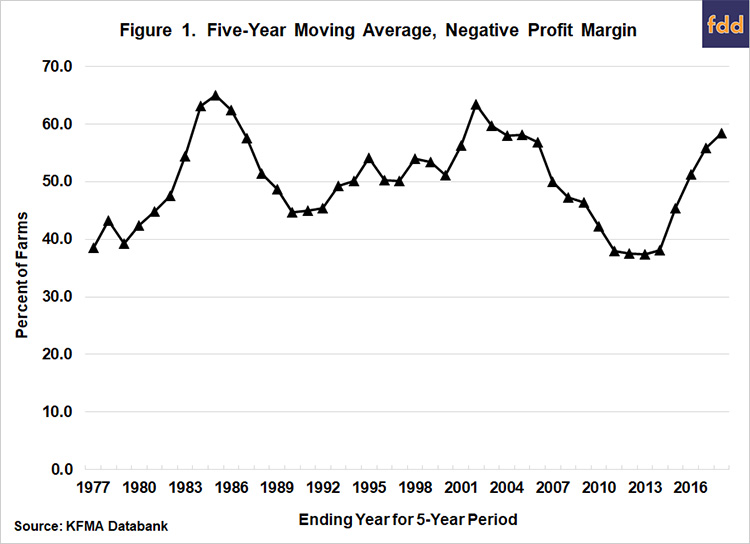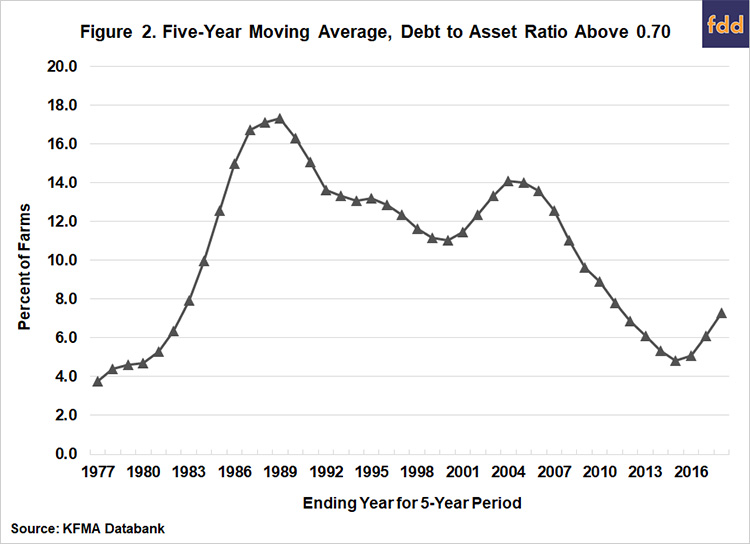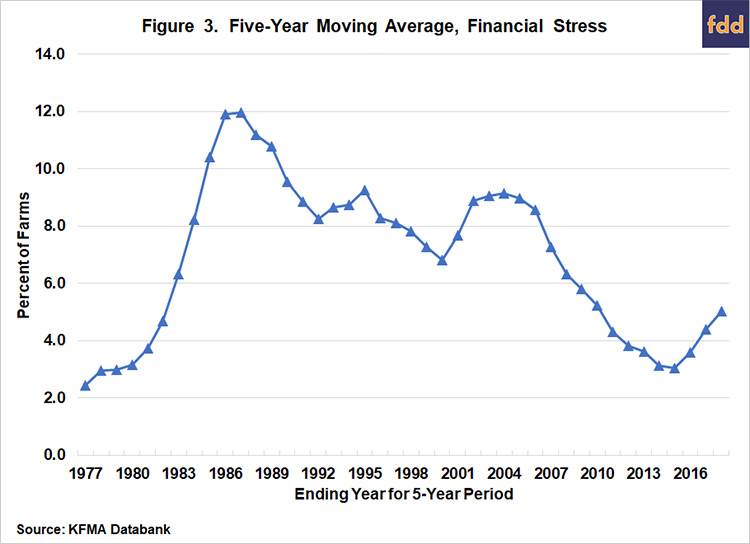# The Incidence of Financial Stress on Kansas Farms, By Michael Langemeier, Center for Commercial Agriculture Purdue University

62recent farmdoc daily (November 1, 2019) article proposed using a negative operating profit margin ratio and a debt to asset ratio above 0.70 to measure whether a farm is financially stressed.  Using this definition of financial stress, this article examines trends in the number of farms with a negative operating profit margin ratio, trends in the number of farms with a debt to asset ratio above 0.70, and trends in financial stress for a sample of Kansas farms.

Whole-farm data for farms participating in the Kansas Farm Management Association (KFMA) were utilized in this article.  Specifically, farms with usable data for individual years from 1973 to 2018 were used to compute the operating profit margin ratio, debt to asset ratio, and the incidence of financial stress.  Rather than reporting annual financial measures, five-year moving averages were utilized.  This data transformation smooths out the annual observations and allows us to focus on long-term trends.

#### Operating Profit Margin Ratio

The operating profit margin ratio is computed by adding interest expense and subtracting unpaid family and operator labor from net farm income and dividing the result by either value of farm production or gross revenue.  A long-run benchmark for the operating profit margin ratio is 20 percent (Langemeier and Yeager, 2018).  Profit margins have been relatively lower than this benchmark during the last few years, particularly for farms with below average long-run performance (e.g., bottom quartile).  For the operating profit margin to be positive, net farm income plus interest expense has to be large enough to cover unpaid family and operator labor.

Figure 1 presents the percentage of KFMA farms with a negative operating profit margin ratio for 5-year periods ending in 1977 through 2018.  Only 6 of the 5-year periods over the 47-year time span, exhibited circumstances in which less than 40 percent of farms did not have a negative operating profit margin ratio.  Ending years for these 6 periods were 1977, 1979, and 2011 to 2014.  The percentage of farms with a negative profit margin has increased dramatically since 2014.  Approximately 58 percent of the farms for the 5-year period ending in 2018 had a negative profit margin.  The percentage of farms with a negative profit margin was higher than the figure for 2018 for the 5-year period ending in 1984, 1985, 1986, 2002, and 2003.#### Debt to Asset Ratio

The debt to asset ratio is computed by dividing total farm debt by total farm assets.  Given the importance of land to total farm assets, land values are an extremely critical determinant of a farm’s debt to asset ratio.  Farms with little to no owned land tend to have relatively higher debt to asset ratios.

The percentages of KFMA farms with a debt to asset ratio above 0.70 for years ending from 1977 to 2018 are reported in Figure 2.  The percentage of high debt farms increased from approximately 3.8 percent of the farms for the 5-year period ending in 1977 to 17.3 percent for the 5-year period ending in 1989, the peak for this ratio.  The percentage of high debt farms reached its second peak for the 5-year period ending in 2004 at 14.1 percent.  The percentage then declined to 4.8 percent in 2015.  Since 2015, the percentage of high debt farms has increased, reaching 7.3 percent for the 5-year period ending in 2018.  The percentage of farms with high debt for the 5-year period ending in 2018 is lower than the percentages exhibited in the 1977 to 1982 5-year periods, and the 2012 to 2017 5-year periods.  Trends in land values are helpful in explaining why the percentage of farms with a high debt to asset ratio is not greater than it currently is.  After almost tripling from 2004 to 2014, land values have declined approximately 12 percent.  More succinctly, land values in 2018 are still more than double what they were in 2006.#### Financial Stress

Farms are said to be financially stressed if the operating margin is relatively low and the debt to asset ratio is relatively high.  Specifically, a farm is said to be financially stressed if the operating profit margin is negative and the debt to asset ratio is above 0.70.  Measuring financial stress is not the same thing as measuring credit quality or the probability of default.  Measures involving credit quality and probability of default typically include the percentage of assets owned, repayment capacity, and working capital (Featherstone and Langemeier, 2017).  Comparing the two concepts, financial stress provides a warning signal whereas credit quality reflects the risk that a farm may not be able to repay short-term and long-term debt.

Figure 3 combines the information in figures 1 and 2, and illustrates the percentage of KFMA farms that were financially stressed for 5-year periods beginning in 1977 and ending in 2018.  Similar to the trend in the percentage of farms with a high debt to asset ratio, the percentage of farms that were financially stressed increased from 2.4 percent in the 5-year period ending in 1977 to 12.0 percent for the 5-year period ending in 1987.  Incidence of financial stress dropped below 10 percent for the 5-year period ending in 1990 and has not come close to reaching this level since.  The percentage was only about 3 percent for the 5-year periods ending in 2014 and 2015.  Even though the incidence of financial stress has increased since 2015, it is still below all of the 5-year periods except the 1977 to 1982 periods and 2011 to 2017 periods.Having a negative profit margin and a high debt to asset ratio were both important determinants of financial stress from 1973 to 2018, however, it is important to note that a high debt to asset ratio explained over 80 percent of the variation in financial stress.  This again points out the importance of trends in land values.  If land values had adjusted more to lower profit margins since 2014, the incidence of financial stress would have been substantially higher in 2018.